RRB JE CE (CBT I) Mock Test- 6

# RRB JE CE (CBT I) Mock Test- 6 - Civil Engineering (CE)

Test Description

## 100 Questions MCQ Test RRB JE Mock Test Series for Civil Engineering (CE) - RRB JE CE (CBT I) Mock Test- 6

RRB JE CE (CBT I) Mock Test- 6 for Civil Engineering (CE) 2023 is part of RRB JE Mock Test Series for Civil Engineering (CE) preparation. The RRB JE CE (CBT I) Mock Test- 6 questions and answers have been prepared according to the Civil Engineering (CE) exam syllabus.The RRB JE CE (CBT I) Mock Test- 6 MCQs are made for Civil Engineering (CE) 2023 Exam. Find important definitions, questions, notes, meanings, examples, exercises, MCQs and online tests for RRB JE CE (CBT I) Mock Test- 6 below.
Solutions of RRB JE CE (CBT I) Mock Test- 6 questions in English are available as part of our RRB JE Mock Test Series for Civil Engineering (CE) for Civil Engineering (CE) & RRB JE CE (CBT I) Mock Test- 6 solutions in Hindi for RRB JE Mock Test Series for Civil Engineering (CE) course. Download more important topics, notes, lectures and mock test series for Civil Engineering (CE) Exam by signing up for free. Attempt RRB JE CE (CBT I) Mock Test- 6 | 100 questions in 90 minutes | Mock test for Civil Engineering (CE) preparation | Free important questions MCQ to study RRB JE Mock Test Series for Civil Engineering (CE) for Civil Engineering (CE) Exam | Download free PDF with solutions
 1 Crore+ students have signed up on EduRev. Have you?
RRB JE CE (CBT I) Mock Test- 6 - Question 1

### Solve the following expression: 452 - 35 * 68 - 58 + 60% of 730.

Detailed Solution for RRB JE CE (CBT I) Mock Test- 6 - Question 1
= 452 - 35 * 68 - 58 + 60% of 730

= 2025 - 2380 - 58 + 438

= 2463 - 2438

= 25

= 52

RRB JE CE (CBT I) Mock Test- 6 - Question 2

### The angles of elevation of the top of a tower 90 m high, from two points on the level ground on its opposite sides are 45° and 60°. What is the distance between the two points (rounded off to the nearest integer)?(√3 = 1.732)

Detailed Solution for RRB JE CE (CBT I) Mock Test- 6 - Question 2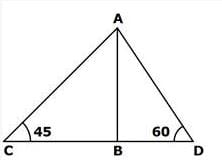Let AB be the tower and C and D the two points.

Now AB/BC=tan⁡45=1

AB=BC=90

Also AB/BD=tan⁡60=√3

BD=90/√3=30√3

Hence CD = CB = 90 + 30√3 = 90 + 51.96 = 141 = 142m

RRB JE CE (CBT I) Mock Test- 6 - Question 3

### Two quadratic equations: 16x2 - Ax + 25 and Bx2 + Ax + 4 = 0 have real and equal roots, then what is the value of √B?

Detailed Solution for RRB JE CE (CBT I) Mock Test- 6 - Question 3
16x2 - Ax + 25 has real and equal roots

(-A)2 = 4 * 16 * 25

A = 40

Bx2 + Ax + 4 = 0 has real and equal roots

(A)2 = 4 * B * 4

(40)2 = 4 * B * 4

B = 100

√B = √100

√B = 10

RRB JE CE (CBT I) Mock Test- 6 - Question 4

Roshan deposited Rs 17500 in the bank which offers compound interest at 8% per annum. What is the interest obtained on the sum after 2 years?

Detailed Solution for RRB JE CE (CBT I) Mock Test- 6 - Question 4
Amount after two years = 17500 x (1+8/100) 2

= 17500 x 1.08 x 1.08 = 20412

Interest = 20412 - 17500 = Rs 2912

RRB JE CE (CBT I) Mock Test- 6 - Question 5

Length of a rectangle is increased by 10%,, and breadth is decreased by 10%. Find the percent effect in the area.

Detailed Solution for RRB JE CE (CBT I) Mock Test- 6 - Question 5
Let length = l

Area = lb

After increment, length = l x (100 + 10)/100 = l x 110/100 = l x 11/10

After decrement, breadth = b x (100 - 10)/100 = b x 90/100 = b x 9/10

Area = l x 11/10 x b x 9/10

= 99lb/100 lb

% decrease = (lb - 99lb/100)/lb x 100

= (100lb - 99lb)/100 x 1/lb x 100

= lb/100 x 1/lb x 100

= 1%

RRB JE CE (CBT I) Mock Test- 6 - Question 6

One of the angles of a triangle is half the larger angle of a parallelogram. The respective ratio between the adjacent angles of the parallelogram is 14 : 31 . The smallest angle of the triangle is half the smaller angle of the parallelogram. What is the value of the largest angle of the triangle?

Detailed Solution for RRB JE CE (CBT I) Mock Test- 6 - Question 6
We know that the sum of the adjacent angles of a parallelogram = 180o

Let the adjacent angles of the parallelogram be 14x and 31x

Therefore, 14x + 31x = 180o

45x = 180o

X = 4

RRB JE CE (CBT I) Mock Test- 6 - Question 7

Aman donated 10% of his Savings to Charity and gave 15% of his savings to his sister. Of the remaining savings his gave 60% to his son and gave 80% of the still remaining saving to his daughter. If the sum of the money given to the sister and the money left with Aman finally is Rs 84,000, what was his savings?

Detailed Solution for RRB JE CE (CBT I) Mock Test- 6 - Question 7
Let his savings be Rs 100x

Money donated to charity = Rs 10x and Money given to sister = Rs 15x

Money left with Aman = 100x - 10x - 15x = Rs 75x

Money left with him after giving to son = (40/100)*75x = Rs 30x

Money left with him after giving to daughter = (20/100)*30x = Rs 6x

Given, 15x + 6x = 84000

=> 21x = 84000

=> x = 4000

His Savings = Rs 4,00,000

RRB JE CE (CBT I) Mock Test- 6 - Question 8

The ratio of 4th and 2nd term of an A.P of positive terms is 5:3, and the product of the 1st and the 3rd terms is 72. what is the product of the 5th and 6th terms of the AP?

Detailed Solution for RRB JE CE (CBT I) Mock Test- 6 - Question 8
Let the first term be 'a' and the common difference be 'd'.

Given, (a + 3d)/(a + d) = 5/3

=> 3a + 9d = 5a + 5d

=> 2a = 4d

=> a = 2d

Also, a(a + 2d) = 72

=> 2d(2d + 2d) = 72

=> 8d2 = 72

=> d2 = 9

=> d = 3

∴ a = 2*3 = 6

d ≠ −3 as the 1st term will become a = 2(−3) = − 6 but all terms are positive.

5th term = a + 4d = 6 + 4*3 = 18

6th term = 18 + 3 = 21

Required product = 18*21 = 378

RRB JE CE (CBT I) Mock Test- 6 - Question 9

Three pipes A, B and C can fill a cistern in 10 hrs. After working together for 4 hours, C is closed and A and B fill the cistern in 9 hrs. Then find the time in which the cistern can be filled by pipe C?

Detailed Solution for RRB JE CE (CBT I) Mock Test- 6 - Question 9
A, B and C can fill in 1 hour = 1/10 of the cistern

A, B, and C can fill in 4 hour= 4/10 =2/5 of the cistern

Remaining part = 1 - 2/5 =3/5 of cistern

3/5 of the cistern is filled by A+B in 9 hours

Therefore, A and B can fill the cistern in 9*5/3 = 15 hrs

Hence in one hour C filled the cistern = 1/10 - 1/15 = 1/30 of the cistern

Hence C can fill the cistern in 30 hrs.

RRB JE CE (CBT I) Mock Test- 6 - Question 10

If the average of twelve consecutive even numbers be 113, then find the sum of the 3rd and 8th numbers among them?

Detailed Solution for RRB JE CE (CBT I) Mock Test- 6 - Question 10
Let the 1st number = x

According to question,

x + (x+2) + (x+4) + ...... + (x+22) = 113*12

12x + 132 = 1356

12x = 1224

x = 102

Therefore 3rd number = 102 + 4 = 106

8th number = 102 + 14 = 116

Hence the required answer = 106 + 116 = 222

RRB JE CE (CBT I) Mock Test- 6 - Question 11

What approximate value should come in the place of the question mark in the following question?

(72)2 ÷ ∛46656=?

Detailed Solution for RRB JE CE (CBT I) Mock Test- 6 - Question 11
? = (72)2 ÷ ∛46656

? = (72)2 ÷ ∛(36x36x36) = (72)2 ÷ 36

? = (72 X 72)/36 = 144

RRB JE CE (CBT I) Mock Test- 6 - Question 12

Ranjan purchases an article at Rs.660 and marks up the price by 25%, and after allowing the discount of Rs.99, he gains __% profit on the sale.

Given below are the steps involved. Arrange them in sequential order.

A) Per cent profit = (66/660) * 100 = 10%

B) Selling price of the item after discount of Rs.99 = 825 - 99 = Rs.726

C) Profit amount earned on the item = 726 - 660 = Rs.66

D) Cost price of the article is Rs.660 and marked price = 125% of 660 = Rs.825

Detailed Solution for RRB JE CE (CBT I) Mock Test- 6 - Question 12
The correct arrangement is

D) Cost price of the article is Rs.660 and marked price = 125% of 660 = Rs.825

B) Selling price of the item after discount of Rs.99 = 825 - 99 = Rs.726

C) Profit amount earned on the item = 726 - 660 = Rs.66

A) Per cent profit = (66/660) * 100 = 10%

RRB JE CE (CBT I) Mock Test- 6 - Question 13

Study the following table carefully and answer the related question.

The following,table represents the three types of products A, B and C manufactured in a company during Five months.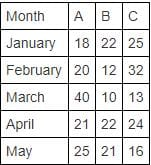In which of the following month there is a maximum percentage increase/decrease in the manufacturing of all three types of products together?

Detailed Solution for RRB JE CE (CBT I) Mock Test- 6 - Question 13
Total products manufactured

In January = 18 + 22 + 25 = 65

In February = 20 + 12 + 32 = 64

In March = 40 + 10 + 13 = 63

In April = 21 + 22 + 24 = 67

In May = 25 + 21 + 16 = 62

Percentage increase/decrease:

In February = ((65 - 64)/65) x 100 = 1.54%

In March = ((64 - 63)/64) x 100 = 1.56%

In April = ((67 - 63)/63) x 100 = 6.35%

In May = ((67 - 62)/67) x 100 = 7.46%

RRB JE CE (CBT I) Mock Test- 6 - Question 14

Two trains 140 m and 160 m long run at the speed of 60 kmph and 40 kmph respectively in opposite directions on parallel tracks. The time (in seconds) which they take to cross each other is :

Detailed Solution for RRB JE CE (CBT I) Mock Test- 6 - Question 14
time = Distance / Velocity

Distance =140+160=300m=0.3km

Relative velocity =60+40=100kmph (opp. direction) Time

=(0.3/100)h

or (0.3/100)×3600=10.8sec

Time =10.8 sec.

RRB JE CE (CBT I) Mock Test- 6 - Question 15

a2+b2=5 and a3+b3=9. If ab = 2, what is the value of a4+b4?

Detailed Solution for RRB JE CE (CBT I) Mock Test- 6 - Question 15
Let (a+b) = c

c2 = (a+b)2 = a2+b2 + 2ab = 5 + 4 = 9

=> a + b = 3

Now, (a+b)(a3+b3) = a4+b4+ab(a2+b2)

=> 3 x 9 = a4+b4 + 2 x 5

=> a4+b4 = 17

RRB JE CE (CBT I) Mock Test- 6 - Question 16

The average weight of 24 students in a class is 40 kg. if the weight of the teacher is included, the average is increased by 500 gms. Find The weight of the teacher?

Detailed Solution for RRB JE CE (CBT I) Mock Test- 6 - Question 16
25*40.5 - 24*40 = 52.5

Hence, the answer is option (2).

RRB JE CE (CBT I) Mock Test- 6 - Question 17

A shopkeeper makes a profit of 20% by selling a T.V. What would be the new profit percent if the shopkeeper paid 15% less for the T.V and the customer paid 10% more for the T.V?

Detailed Solution for RRB JE CE (CBT I) Mock Test- 6 - Question 17
Let the price at which T.V was purchased by shopkeeper = Rs 100a

Hence the price at which T.V was sold by shopkeeper = Rs 120a

If shopkeeper paid 15% less than the previous amount hence new cost price of the T.V = Rs (100a X 0.85) = Rs 85a

New selling price of the T.V = Rs (120a X 1.1) = Rs 132a

Profit % = (132a-85a)/85a X 100 = 55.3%

RRB JE CE (CBT I) Mock Test- 6 - Question 18

Solve the following expression: (13/8) of (15/32) of 45% of 3072.

Detailed Solution for RRB JE CE (CBT I) Mock Test- 6 - Question 18
= (13/8) of (15/32) of 45% of 3072

= (13/8) * (15/32) * (45/100) * 3072

= (13/8) * (3/32) * (9/4) * 307

= 13 * 3 * 9 * 3

= 1053

RRB JE CE (CBT I) Mock Test- 6 - Question 19

Following Pie Chart shows the percentage distribution of people of different ages in a Building. The table shows the ratio of Male and Female people in each age group. There is a total of 800 people in the building.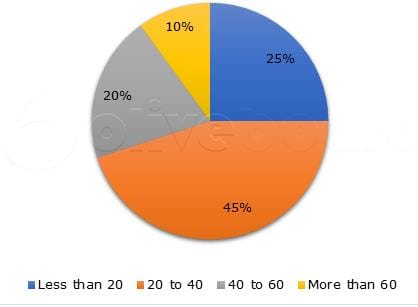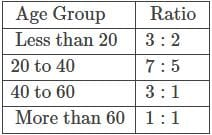What is the difference between the total number of Males of age 20 to 60 and the total number of Females of age 20 to 60?

Detailed Solution for RRB JE CE (CBT I) Mock Test- 6 - Question 19
Calculating the number of Male and Females of each age group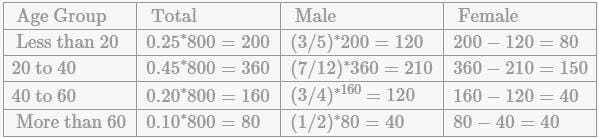total number of Males of age 20 to 60 = 210 + 120 = 330

total number of Females of age 20 to 60 = 150 + 40 = 190

Required difference = 330 - 190 = 140

RRB JE CE (CBT I) Mock Test- 6 - Question 20

The population of a village is 6000. The next year, the number of male increases by 10% and the number of female increases by 20% and the population becomes 6840. What is the initial population of Male?

Detailed Solution for RRB JE CE (CBT I) Mock Test- 6 - Question 20
Let the initial population of Males and Females be 100x and 100y respectively.

Given, 100x + 100y = 6000

=> x + y = 60..(i)

And, 110x + 120y = 6840

=> 11x + 12y = 684..(ii)

12*(i) - (ii), gives

x = 36

Initial population of Males = 3600

RRB JE CE (CBT I) Mock Test- 6 - Question 21

The average age of a Sheela and her sister is 16 years. The respective ratio of their ages is 7:1 respectively. Find Sheela's age?

Detailed Solution for RRB JE CE (CBT I) Mock Test- 6 - Question 21
Sum of their ages = 2 X 16 = 32

Let 7x and x be their respective ages, then, 8x = 32 and x = 4

So, Sheela's age = 7x = 7 X 4 = 28 years . Hence, option 1.

RRB JE CE (CBT I) Mock Test- 6 - Question 22

20% of x% of 60 is equal to y% of 60% of 40, what is the value of the ratio x:y?

Detailed Solution for RRB JE CE (CBT I) Mock Test- 6 - Question 22
20% of x% of 60 = y% of 60% of 40

x = 2y

x:y = 2:1

RRB JE CE (CBT I) Mock Test- 6 - Question 23

What is the remainder when x20 + x19 + x18 + x17 + x16 + x15 + x14 + x13 + x12 + x11 is divided by x9 + x8 + x7 + x6 + x5 + x4 + x3 + x2 + x + 1

Detailed Solution for RRB JE CE (CBT I) Mock Test- 6 - Question 23
Numerator = x11 (x9 + x8 + x7 + x6 + x5 + x4 + x3 + x2 + x + 1) = x11 times the denominator.

The denominator divides the numerator, so the answer is 0.

RRB JE CE (CBT I) Mock Test- 6 - Question 24

A person invested equal amounts in two scheme A and B at the same rate of interest. Scheme A offers simple interest while scheme B offers compound interest. After two years he got Rs. 1920 from the Scheme A as interest and Rs. 2112 from scheme B. If the rate of interest is increased by 4%, what will be the total interest after two years both schemes ?

Detailed Solution for RRB JE CE (CBT I) Mock Test- 6 - Question 24
C.I−S.I=192

S.I for 1 year =960 Interest on RS960 for 1 year is 192 so rate is =(192×100)/(960×1)=20%

so the principal is =(960×100)/20=4800 when interest is increased is 4% then new rate of interest is 24% So simple interest after two years will be = (4800×2×24)/100=2304 and compound interest rate after two years will be =24+24+(24×24)/100=53.76% Compound interest after two years =(53.76×4800)/100=2580.48 So total interest is = 2304 + 2580.48 = Rs. 4884.48

RRB JE CE (CBT I) Mock Test- 6 - Question 25

How many litres of a solution containing milk and water in the ratio 2:3 should be added to 30 litres of 70 % milk solution in order to get 60% milk solution?

Detailed Solution for RRB JE CE (CBT I) Mock Test- 6 - Question 25
Let the number of liters of solution added be: x

The initial ratio of milk (m) to water (w) is:

=> m/w = 2/3

=> m = 2/5 of the solution

=> m = 40% of the solution

Acc. to the question, after adding x liters of 40% milk solution to 30 liters of 70% milk solution, we get 60% of (x+30) liters milk solution.

Hence, the equation becomes:

=> 40x + 30 * 70 = (x+30) * 60

=> 40x + 2100= 60x + 1800

=> 20x = 300

=> x = 15

RRB JE CE (CBT I) Mock Test- 6 - Question 26

If 12% of a is equal to 15% of b. Find the respective ratio of a and b.

Detailed Solution for RRB JE CE (CBT I) Mock Test- 6 - Question 26
12% of a = 15% of b

=> a/b = 15/12

=> a/b = 5/4

=> a:b = 5:4

RRB JE CE (CBT I) Mock Test- 6 - Question 27

Downstream speed of boat A is 20% more than the downstream speed of boat B whose upstream speed is 6 km/hr less than upstream speed of boat A. What is the speed of boat A in still water? The stream for both boat is moving with 10 km/hr.

Detailed Solution for RRB JE CE (CBT I) Mock Test- 6 - Question 27
Stream speed = 10 km/hr

Downstream speed of boat A = (6/5) x downstream speed of boat B

Upstream speed of boat A - upstream speed of boat B = downstream speed of boat A - downstream speed of boat B = 6

(6/5) x downstream speed of boat B - downstream speed of boat B = 6

Downstream speed of boat B = 30 km/hr

Downstream speed of boat A = 30 + 6 = 36 km/hr

Speed of boat A in still water = 36 - 10 = 26 km/hr

RRB JE CE (CBT I) Mock Test- 6 - Question 28

If the the ,monthly income of a person is increased by 20% and his expenditures are increased by 40%, then his savings are decreased by what percent? (ratio of income and expenditure is 4: 3 respectively.)

Detailed Solution for RRB JE CE (CBT I) Mock Test- 6 - Question 28
Let income and expenditure are 'a' and 'b' respectively.

Savings = a - b = a - 3a/4 = a/4

When income and expenditure are increased by 20%. Then,

New income = 120% of a = 1.2a

New expenditure = 140% of b = 1.4 x 3a/4 = 2.1a/2

New savings = 1.2a - 2.1a/2 = 3a/20

Decrement in savings = (a/4) - (3a/20) = a/10

Percentage = a/10)/(a/4 x 100 = 40%

RRB JE CE (CBT I) Mock Test- 6 - Question 29

A and B can finish a work in 2 days, B and C in 3 days and A and C in 4 days. Find the ratio of time taken by A alone and C alone to finish the work?

Detailed Solution for RRB JE CE (CBT I) Mock Test- 6 - Question 29
Suppose A, B and C take A, B and C days respectively to finish the work on their own.

1/A + 1/B = 1/2 (1)

1/B + 1/C = 1/3 (2)

1/A + 1/C = 1/4 (3)

(1)+(2)+(3) => 2(1/A+1/B+1/C) = 13/12

=> 1/A + 1/B + 1/C = 13/24 (4)

(4) - (2) => 1/A = 5/24

Thus A takes 24/5 = 4.8 days.

(4) - (1) => 1/C = 1/24

Hence C takes 24 days.

Required ratio = 4.8: 24 = 1:5

RRB JE CE (CBT I) Mock Test- 6 - Question 30

Which of the following is irrational?

Detailed Solution for RRB JE CE (CBT I) Mock Test- 6 - Question 30
Product of (3 - √27) and (√9 + 3√3)

= (3 - 3√3)(3 + 3√3)

= - 18 (which is not irrational)

Option (2):

= Sum of (5 - 4√2) and 4(6 + √2)

= (5 - 4√2) + 4(6 + √2)

= 5 - 4√2 + 24 + 4√2

= 29 (which is not irrational)

Option (3):

= Sum of √4761 and √5175

= 69 + 15√23 (which is irrational)

Option (4):

= Product of √16875 and √27

= 75√3 x 3√3

= 675 (which is not irrational)

RRB JE CE (CBT I) Mock Test- 6 - Question 31

Pointing to a boy in a photograph Akhil said "He is the son of the only son of my mother". How is Akhil related to that boy?

Detailed Solution for RRB JE CE (CBT I) Mock Test- 6 - Question 31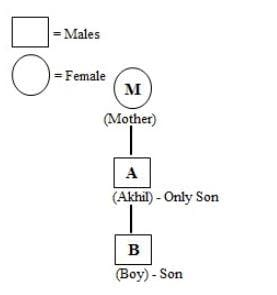RRB JE CE (CBT I) Mock Test- 6 - Question 32

If’ A’ is coded as 1, ‘B’ as 3, ‘C’ as 5 and so on, which of the following is the numerical value of the word ‘FAZED’?

Detailed Solution for RRB JE CE (CBT I) Mock Test- 6 - Question 32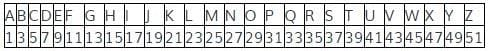So from the above table, numerical value of FAZED = 11 + 1 + 51 + 9 + 7 = 79.

RRB JE CE (CBT I) Mock Test- 6 - Question 33

DIRECTIONS: In the given question find the answer figure in which question is embedded.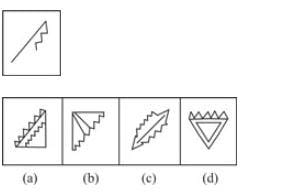Detailed Solution for RRB JE CE (CBT I) Mock Test- 6 - Question 33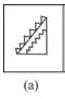Hence option A is the right answer.

RRB JE CE (CBT I) Mock Test- 6 - Question 34

DIRECTIONS: Read the following information carefully to answers the questions that follow.

Two brothers Manin and Nitin walk in opposite directions for 3km. Manin is walking towards East.After 3km each, both turn right and again walk 3 km each. Both turn to face each other.

In which direction is Nitin looking?

Detailed Solution for RRB JE CE (CBT I) Mock Test- 6 - Question 34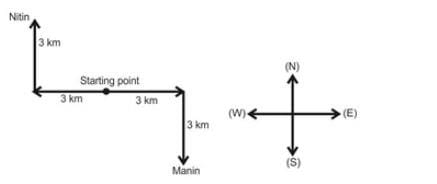From the above diagram, it is clear that, Nitin is looking in South-East direction.

RRB JE CE (CBT I) Mock Test- 6 - Question 35

In the given sequence, how many such symbols and numbers are there which are either immediately preceded or immediately followed by the letters which is from the first half of the English alphabet?

2 L K @ 8 \$ P B 1 V # 6 % G W 9 J C D 4 © 7 F R 4 A

Detailed Solution for RRB JE CE (CBT I) Mock Test- 6 - Question 35
Such combinations are

2L, K@, B1, %G, 9J, D4, 7F, 4A,

RRB JE CE (CBT I) Mock Test- 6 - Question 36

Select the odd letter/word/number from the given alternatives.

Detailed Solution for RRB JE CE (CBT I) Mock Test- 6 - Question 36
All others are the drinks.
RRB JE CE (CBT I) Mock Test- 6 - Question 37

DIRECTIONS: Each of the following questions consists of five figures marked A, B, C, D and E if called the Problem figures followed by five other figures marked 1, 2, 3 and 4 called the Answer Figures. Select a figure from amongst the Answer Figures which will continue the same series as established by the five Problem Figures.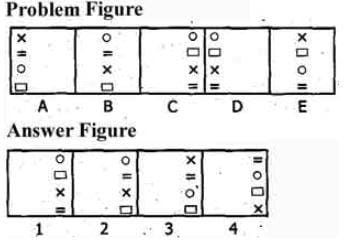Detailed Solution for RRB JE CE (CBT I) Mock Test- 6 - Question 37
All the symbols move horizontally. In the first step, the first and third symbols interchange positions. In the second step, the second and fourth symbols interchange positions. In the third step, the symbols remain as such. The Whole process is then repeated.
RRB JE CE (CBT I) Mock Test- 6 - Question 38

DIRECTIONS: Each of the following questions consists of five figures marked A, B, C, D and E called the Problem Figures followed by five other figures marked 1, 2, 3 and 4 called the Answer figures. Select a figure from amongst the Answer Figures which will continue the same series as established by the five Problem Figures.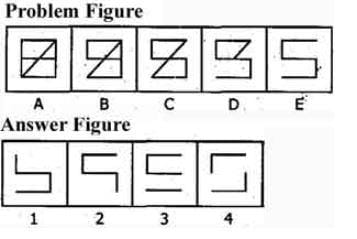Detailed Solution for RRB JE CE (CBT I) Mock Test- 6 - Question 38
One line segment disappears from the figure In each step, one from the lower portion and one from the upper portion alternately.
RRB JE CE (CBT I) Mock Test- 6 - Question 39

Choose the odd one out from the given alternatives.

Detailed Solution for RRB JE CE (CBT I) Mock Test- 6 - Question 39
First number ÷ Second number = Remainder 2

26/3 = Remainder 2

39/4 = Remainder 3

42/5= Remainder 2

44/6 = Remainder 2

RRB JE CE (CBT I) Mock Test- 6 - Question 40

In a certain code language, ‘nick muk pic’ means ‘brave and concern’, ‘ill dic so’ means ‘every body else’ and ‘tur muk so’ means ‘body and soul’. Which of the following may mean ‘and every soul’?

Detailed Solution for RRB JE CE (CBT I) Mock Test- 6 - Question 40
From (1) & (3)

muk = and

From (2) & (3)

so = body

From (3)

soul = tur

From (2)

every / else = ill/dic

From (1)

concern/brave = nick / pic

So, ‘and every soul’ may be ‘muk tur dic’,.

RRB JE CE (CBT I) Mock Test- 6 - Question 41

Rajitha walked 80 m before taking a left turn. She then walked 60 m and took a left turn to walk another 50 m. Finally she took a right turn and walked 30 m to reach the bank, now if she faces East, in which direction did she start walking?

Detailed Solution for RRB JE CE (CBT I) Mock Test- 6 - Question 41
The path traced by Rajitha is as follows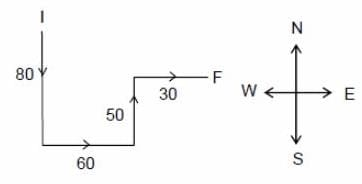As Rajitha was facing East at the end which implies that initially she started walking in the south direction.

RRB JE CE (CBT I) Mock Test- 6 - Question 42

DIRECTIONS: In each of the following questions, choose the set of numbers out of the four options that is similar to the given set.

given set: (21, 51, 15)

Detailed Solution for RRB JE CE (CBT I) Mock Test- 6 - Question 42
Middle numbers = first number + 2 × second number.
RRB JE CE (CBT I) Mock Test- 6 - Question 43

DIRECTIONS: In each of the following questions, choose the set of numbers out of the four options that is similar to the given set.

Given set : (63, 8, 3)

Detailed Solution for RRB JE CE (CBT I) Mock Test- 6 - Question 43
Add 1 to the first number and take the square root to find the second number, and similarly with the given the wordgiven the wordgiven the wordnd number we will find 3rd number
RRB JE CE (CBT I) Mock Test- 6 - Question 44

DIRECTIONS: In the following question, continuous pattern series is given. Some of the letters of the series are missing. These missing letters are given in that order as one of the four alternatives below the series. Find out the correct alternatives.

Detailed Solution for RRB JE CE (CBT I) Mock Test- 6 - Question 44
In the above series, the letters are equidistant from the beginning and the end.

Hence, the missing letter are cbbaa.

RRB JE CE (CBT I) Mock Test- 6 - Question 45

DIRECTIONS: In the question below, there are few statements followed by few conclusions. You have to take the given statements to be true even if they seem to be at variance with commonly known facts and then decide which of the given conclusion logically follow(s) from the given statements.

Statements: All fruits are lions.

All lions are foxes.

Some foxes are elephants.

Conclusions:

I. All fruits are foxes.

II. Some fruits are elephants.

Detailed Solution for RRB JE CE (CBT I) Mock Test- 6 - Question 45
Only Conclusion I follows.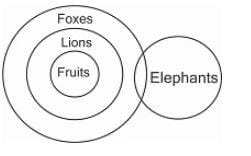RRB JE CE (CBT I) Mock Test- 6 - Question 46

DIRECTIONS: Study the venn diagram to answer the given question.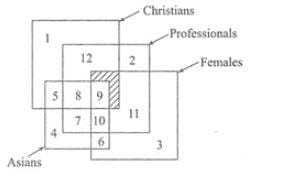Asian non-Christian females who are professionals are represented by-

Detailed Solution for RRB JE CE (CBT I) Mock Test- 6 - Question 46Hence option B is the right answer.

RRB JE CE (CBT I) Mock Test- 6 - Question 47

Pointing to a man in the photograph a woman says: “He is the father of my daughter-in-law’s brother-in-law.” How is the man related to the woman?

Detailed Solution for RRB JE CE (CBT I) Mock Test- 6 - Question 47
Daughter-in-law’s brother-in-law = Son.

Father of son = Husband.

RRB JE CE (CBT I) Mock Test- 6 - Question 48

If B is coded as 8, F is coded as 6, Q is coded as 4, D is coded as 7, T is coded as 2, M is coded as 3, and K is coded as 5, then what is the coded form of QKTBFM?

Detailed Solution for RRB JE CE (CBT I) Mock Test- 6 - Question 48
Q K T B F M = 4 5 2 8 6 3
RRB JE CE (CBT I) Mock Test- 6 - Question 49

Which of the words in the following options can be formed using the given word ?

DETERMINATION

Detailed Solution for RRB JE CE (CBT I) Mock Test- 6 - Question 49
There is no ‘C’ in the given word.So, DECLARATION cannot be formed.

There is no ‘L’ in the given word.So, NATIONAL cannot be formed.

There is no ‘V’ in the given word.So, DEVIATION cannot be formed.

RRB JE CE (CBT I) Mock Test- 6 - Question 50

DIRECTIONS: Arrange the following in a logical order.

1. Puberty

3. Childhood

4. Infancy

5. Senescence

Detailed Solution for RRB JE CE (CBT I) Mock Test- 6 - Question 50
4. Infancy

3. Childhood

1. Puberty

5. Senescence

Hence option B is the right option.

RRB JE CE (CBT I) Mock Test- 6 - Question 51

Cattle, cows and oxen can be represented by

Detailed Solution for RRB JE CE (CBT I) Mock Test- 6 - Question 51
Cow and oxen both are cattle.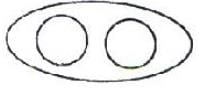RRB JE CE (CBT I) Mock Test- 6 - Question 52

The day on 18.09.1977 was Sunday. A couple was married on this date. In the next 15 years, there how many marriage anniversaries would fall on Sunday?

Detailed Solution for RRB JE CE (CBT I) Mock Test- 6 - Question 52
1977 is an ordinary year. We know that the calendar of an ordinary year repeats after 6 yrs or 11 yrs.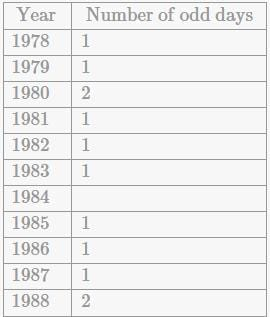Number of odd days from 18.09.1977 to 18.09.1983=7, i.e., 0 odd day

It means that in 1983, 18th September would fall on Sunday.

and number of odd days from 18.09.1977 to 18.09.1988 = 14, i.e., 0 odd day.

Now, it is clear that 2 marriage anniversaries would fall on Sunday in the next 15 yr.

RRB JE CE (CBT I) Mock Test- 6 - Question 53

In a queue, Lily is eighteenth from the front while Mini is sixteenth from the back .If Nisha is twenty- fifth from the front and is exactly in the middle of Lily and Mini, then how many persons are there in the queue?

Detailed Solution for RRB JE CE (CBT I) Mock Test- 6 - Question 53
Lily is 18th from front and Nisha is 25th .

Number of persons between Lily and Nisha = 6.

Since Nisha is exactly in middle of Lily and Mini, so number of persons between Nisha and Mini = 6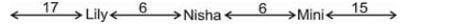∴ Number of persons in the queue = (17 + 1 + 6 + 1 + 6 + 1 + 15) = 47.

RRB JE CE (CBT I) Mock Test- 6 - Question 54

DIRECTIONS: In the question below, which character when placed at the sign of interrogation shall complete the matrix?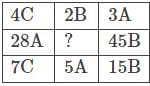Detailed Solution for RRB JE CE (CBT I) Mock Test- 6 - Question 54
In each row, out of the letters A, B and C, each of these must appear once. In each column, the product of the first and third numbers is equal to the second number. So, the missing number will be (2 × 5) i.e., 10 and the letter will be C.

RRB JE CE (CBT I) Mock Test- 6 - Question 55

DIRECTIONS : Given below are two Matrices of Twenty-five Cells, each containing two classes of alphabets. The columns and rows of Matrix I are numbered form 0 to 4 and that of Matrix II from 5 to 9. A letter from these Matrices can be represented first by its row number and next by its column number. In each of the following questions, identify one set of number pairs out of (1), (2), (3) and (4) which represents the given word.

HARD

MATRIX-1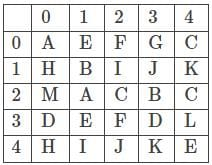MATRIX-2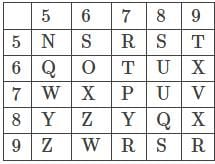Detailed Solution for RRB JE CE (CBT I) Mock Test- 6 - Question 55
HARD

H– 10, 40

A– 00, 21

R– 57, 97, 99

D– 30, 33

Therefore, the correct answer is option (3) i.e. 40, 21, 57, 33

RRB JE CE (CBT I) Mock Test- 6 - Question 56

All India Muslim league was set up in the year_________.

Detailed Solution for RRB JE CE (CBT I) Mock Test- 6 - Question 56
All India Muslim League was set up in the year 1906 at Dhaka.

Founders of All India Muslim League- Khwaja Salimullah, Vikar-ul-Mulk, Syed Amir All, Syed Nabiullah.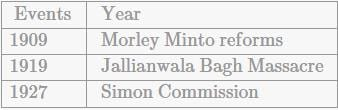RRB JE CE (CBT I) Mock Test- 6 - Question 57

India's First Global Skills Park will be established in__________.

Detailed Solution for RRB JE CE (CBT I) Mock Test- 6 - Question 57
Asian Development Bank (ADB) and the Government of India signed a \$150 million loan agreement to establish a Global Skills Park (GSP) in Madhya Pradesh i.e. first Multi-Skills Park in India, to enhance the quality of technical and vocational education.
RRB JE CE (CBT I) Mock Test- 6 - Question 58

Which of the following countries, recently rolled out the world's first hydrogen-powered train.?

Detailed Solution for RRB JE CE (CBT I) Mock Test- 6 - Question 58
Germany rolled out the world's first hydrogen-powered train. It uses fuel cells that produce electricity through a combination of hydrogen and oxygen, leaving behind water and steam. It is built by French TGV-maker Alstom.
RRB JE CE (CBT I) Mock Test- 6 - Question 59

What was the original name of Nur Jahan?

Detailed Solution for RRB JE CE (CBT I) Mock Test- 6 - Question 59
Noor Jehan (1577-1645) was an empress who belonged to the great Mughal Dynasty. Noor Jehan was the favourite wife of the Mughal Emperor Jahangir. Her real name was Mehr-un-Nisa.
RRB JE CE (CBT I) Mock Test- 6 - Question 60

How many schedules are there in the Indian constitution?

Detailed Solution for RRB JE CE (CBT I) Mock Test- 6 - Question 60
There are twelve schedules in the Indian constitution. Schedules are lists in the constitution which categorise and tabulate bureaucratic activity and government policy. It is an appendix or annexure highlighting lists or details mentioned in its main text.
RRB JE CE (CBT I) Mock Test- 6 - Question 61

__________ five-year plan was based on the P.C Mahalanobis Model.

Detailed Solution for RRB JE CE (CBT I) Mock Test- 6 - Question 61
Second Five-year plan was based on the P.C Mahalanobis Model.

The second Five Year Plan centered on a shift towards developing capital goods and heavy industry for long-term economic benefit. During that period, agriculture spending fell from 37 percent of public spending to 20.9 percent, while industry allocation increased from 4.9 to 24.1 percent.

RRB JE CE (CBT I) Mock Test- 6 - Question 62

Wold Oceans day is observed every year on_______.

Detailed Solution for RRB JE CE (CBT I) Mock Test- 6 - Question 62
Wold Oceans day is observed every year on 8th of June.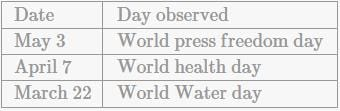RRB JE CE (CBT I) Mock Test- 6 - Question 63

The 6th RCEP Trade Ministers Meeting took place in________

Detailed Solution for RRB JE CE (CBT I) Mock Test- 6 - Question 63
The 6th RCEP Trade Ministers' Meeting took place in Singapore. It consists of 10 ASEAN countries and six ASEAN FTA partners namely, India, China, Japan, Korea, Australia and New Zealand. RCEP-Regional Comprehensive Economic Partnership. Suresh prabhu represented India.
RRB JE CE (CBT I) Mock Test- 6 - Question 64

Who among the following discovered dynamite?

Detailed Solution for RRB JE CE (CBT I) Mock Test- 6 - Question 64
The dynamite was discovered by Alfred Nobel.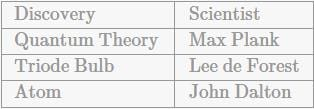RRB JE CE (CBT I) Mock Test- 6 - Question 65

________ clinched the Davis cup, 2018 title.

Detailed Solution for RRB JE CE (CBT I) Mock Test- 6 - Question 65
Marin Cilic clinched Croatia's second Davis Cup title beating France's Lucas Pouille. The 2018 Davis Cup was the 107th edition of the Davis Cup, a tournament between national teams in men's tennis. Croatia clinched the title 13 years after the first title.
RRB JE CE (CBT I) Mock Test- 6 - Question 66

Kalinga cup is associated with the game of ___________.

Detailed Solution for RRB JE CE (CBT I) Mock Test- 6 - Question 66
Kalinga cup is associated with the game of Football.

Duleep trophy- Cricket

Aga Khan Cup- Hockey

RRB JE CE (CBT I) Mock Test- 6 - Question 67

Who among the following has been sworn in as the new president of Brazil?

Detailed Solution for RRB JE CE (CBT I) Mock Test- 6 - Question 67
Jair Bolsonaro has been sworn in as the new president of Brazil after a controversial, polarizing campaign to radically change the center-left path taken by Latin America's biggest country over the last 15 years.
RRB JE CE (CBT I) Mock Test- 6 - Question 68

Who among the following is an author of the book 'War and Peace'?

Detailed Solution for RRB JE CE (CBT I) Mock Test- 6 - Question 68
The book 'War and Peace' has been written by Leo Tolstoy.

Book - Author

The wealth of Nations - Adam Smith

Oliver Twist - Charles Dickens

RRB JE CE (CBT I) Mock Test- 6 - Question 69

In each of the questions below, two statements are given, followed by two assumptions on which the passage may or may not lie. From the options below, choose the one that reflects the correct choice of assumptions that follow.

Ramesh's teachers suspect that he is facing child abuse at home, since his grades have dropped significantly.

I. The home environment of a child has the capacity to affect a child's school grades.

II. Children who face child abuse often fare poorly in academics.

Detailed Solution for RRB JE CE (CBT I) Mock Test- 6 - Question 69
I follows as only if I is assumed, can Ramesh's teachers' suspicion hold true and have substance. II also follows, as if true, then the teachers' suspicion can be said to be valid. C is the right answer.
RRB JE CE (CBT I) Mock Test- 6 - Question 70

______ has been adjudged the most liveable city in 2018.

Detailed Solution for RRB JE CE (CBT I) Mock Test- 6 - Question 70
According to Economist Intelligence Unit's Global Liveability Index, Vienna has been adjudged the most liveable city in 2018 followed by Melbourne. Damascus retained last place, followed by the Bangladeshi capital Dhaka.
RRB JE CE (CBT I) Mock Test- 6 - Question 71

Which vitamin is prepared by our body in the presence of sunlight?

Detailed Solution for RRB JE CE (CBT I) Mock Test- 6 - Question 71
Vitamin D.-Vitamin D can be made by our body itself. Our skin uses sunlight to produce vitamin D.
RRB JE CE (CBT I) Mock Test- 6 - Question 72

Charle’s Law describes the relation between -

Detailed Solution for RRB JE CE (CBT I) Mock Test- 6 - Question 72
Charle’s Law describes the relation between Volume and Temperature
RRB JE CE (CBT I) Mock Test- 6 - Question 73

Where does the oxygen that keeps us alive come form?

Detailed Solution for RRB JE CE (CBT I) Mock Test- 6 - Question 73
Since water and carbon dioxide are both compounds which contain oxygen, it is possible to obtain oxygen from either. The oxygen produced from photosynthesis is not released into the air. It is not until cellular respiration that oxygen is released. the oxygen comes from water. The water molecules are split at the “beginning” of photosynthesis for the electrons. These electrons eventually make their way to the electron transport chain, where oxygen is the final electon accept, and then released into the air. Scientists agree that there’s oxygen from ocean plants in very breath we take. Most of this oxygen comes from tiny ocean plants – called phytoplankton-that live near the water’s surface and drift with the currents. Like all plants, they photosynthesize-that is, they use sunlight and carbon dioxide to make food. A byproduct of photosynthesis is oxygen.
RRB JE CE (CBT I) Mock Test- 6 - Question 74

Gamma rays were discovered by -

Detailed Solution for RRB JE CE (CBT I) Mock Test- 6 - Question 74
It was discovered by Paul Villard.It consists of the shortest wavelength electromagnetic waves and so imparts the highest photon energy. Paul Villard, a French chemist and physicist, discovered gamma radiation in 1900 while studying radiation emitted by radium.
RRB JE CE (CBT I) Mock Test- 6 - Question 75

Which of the following is an example of Newton’s Third Law?

Detailed Solution for RRB JE CE (CBT I) Mock Test- 6 - Question 75
Newton’s Third Law states that “To every action, there is an equal and opposite reaction”. When a gun is fired, it thus recoils and while we swim we exert force on the water which exerts force on us, due to which we move forward.
RRB JE CE (CBT I) Mock Test- 6 - Question 76

J J Thomson is credited with the discovery of -

Detailed Solution for RRB JE CE (CBT I) Mock Test- 6 - Question 76
Sir Joseph John Thomson OM PRS (18 December 1856 – 30 August 1940) was a British physicist and Nobel Laureate in Physics, credited with the discovery of the electron, the first subatomic particle to be discovered.
RRB JE CE (CBT I) Mock Test- 6 - Question 77

Smallpox is caused by:

Detailed Solution for RRB JE CE (CBT I) Mock Test- 6 - Question 77
Smallpox is caused by two virus variants-Variola major and Variola minor.
RRB JE CE (CBT I) Mock Test- 6 - Question 78

The shape of a rain drop is spherical due to

Detailed Solution for RRB JE CE (CBT I) Mock Test- 6 - Question 78
High surface tension binds the raindrop from all corners from inside and that is why the raindrop is spherical in shape
RRB JE CE (CBT I) Mock Test- 6 - Question 79

First biosphere reserve was established is 1986 at-

Detailed Solution for RRB JE CE (CBT I) Mock Test- 6 - Question 79
First biosphere reserve was established is 1986 at Nilgiri.
RRB JE CE (CBT I) Mock Test- 6 - Question 80

A boat will submerge when it displaces water equal to its own _______

Detailed Solution for RRB JE CE (CBT I) Mock Test- 6 - Question 80
A boat will float when the weight of the water it displaces equals the weight of the boat and anything will float if it is shaped to displace its own weight of water before it reaches the point where it will submerge. Floating of the boat works on the principle of buoyancy force which is an upward force exerted by a liquid, gas or other fluid, that opposes the weight of an immersed object. In a column of fluid, pressure increases with depth as a result of the weight of the overlying fluid. Thus a column of fluid, or an object submerged in the fluid, experiences greater pressure at the bottom of the column than at the top. This difference in pressure results in a net force that tends to accelerate an object upwards.
RRB JE CE (CBT I) Mock Test- 6 - Question 81

Which instrument is used to measure wind speed?

Detailed Solution for RRB JE CE (CBT I) Mock Test- 6 - Question 81
Anemometer is a measuring instrument used in meteorology to measure wind speed. The first anemometer was invented by Leon Battista Alberti in 1450.
RRB JE CE (CBT I) Mock Test- 6 - Question 82

Scurvy is caused by the deficiency of?

Detailed Solution for RRB JE CE (CBT I) Mock Test- 6 - Question 82
Scurvy is caused by the deficiency of Vitamin C
RRB JE CE (CBT I) Mock Test- 6 - Question 83

Diamond is -

Detailed Solution for RRB JE CE (CBT I) Mock Test- 6 - Question 83
Diamond is an element.
RRB JE CE (CBT I) Mock Test- 6 - Question 84

Friction is a

Detailed Solution for RRB JE CE (CBT I) Mock Test- 6 - Question 84
The force responsible for changing the state of motion of objects in contact is called the force of friction. It always acts on all moving objects.
RRB JE CE (CBT I) Mock Test- 6 - Question 85

The measurement of the gravitational pull on an object is its

Detailed Solution for RRB JE CE (CBT I) Mock Test- 6 - Question 85
weight-the measurement of the gravitational pull on an object is its weight.
RRB JE CE (CBT I) Mock Test- 6 - Question 86

Two richest known sources of edible protein are _______.

Detailed Solution for RRB JE CE (CBT I) Mock Test- 6 - Question 86
The richest sources of protein are animal foods such as chicken, meat, fish, cheese and eggs. However, plant proteins are believed to be healthier because of their lower fat content. Plant protein is found (e.g.) in beans (esp. soy beans), lentils, nuts, quorn and seeds. Fish and seafood are some of the richest sources of protein. One-half of a typical fillet of halibut or salmon provides approximately 41g of protein. Soybeans are legumes that provide a valuable protein-rich food option for vegetarians and non-vegetarians alike. One cup of boiled soybeans provides over 28g of protein.
RRB JE CE (CBT I) Mock Test- 6 - Question 87

Modulus of elasticity is defined as the ratio of:

Detailed Solution for RRB JE CE (CBT I) Mock Test- 6 - Question 87
Modulus of elasticity is defined as the ratio of Linear stress to linear strain
RRB JE CE (CBT I) Mock Test- 6 - Question 88

NSC (National Savings Certificates) are issued in the denominations of :

Detailed Solution for RRB JE CE (CBT I) Mock Test- 6 - Question 88
National Savings Certificates can be issued in denominations of Rs. 100, Rs. 500, Rs. 1000, Rs.
RRB JE CE (CBT I) Mock Test- 6 - Question 89

Which of the following is a Compound?

Detailed Solution for RRB JE CE (CBT I) Mock Test- 6 - Question 89
Sulphur and Gold are element while air is mixture of various compound made of element Hydrogen and Oxygen and its Chemical formula is H2O.
RRB JE CE (CBT I) Mock Test- 6 - Question 90

Which of the following is a vector?

Detailed Solution for RRB JE CE (CBT I) Mock Test- 6 - Question 90
Displacement is a vector quantity while the rest are scalar
RRB JE CE (CBT I) Mock Test- 6 - Question 91

Who invented the aeroplane?

Detailed Solution for RRB JE CE (CBT I) Mock Test- 6 - Question 91
The Wright Brothers had invented the aeroplane.
RRB JE CE (CBT I) Mock Test- 6 - Question 92

For a missile launched with a velocity less than the earth’s escape velocity, the total energy is

Detailed Solution for RRB JE CE (CBT I) Mock Test- 6 - Question 92
When a missile is launched with a velocity less than the escape velocity of the earth, the sum of its kinetic energy and potential energy is negative.
RRB JE CE (CBT I) Mock Test- 6 - Question 93

What is the atomic number of Iron?

Detailed Solution for RRB JE CE (CBT I) Mock Test- 6 - Question 93
The atomic number of Iron is 26.
RRB JE CE (CBT I) Mock Test- 6 - Question 94

Which one of the following has greatest mass?

Detailed Solution for RRB JE CE (CBT I) Mock Test- 6 - Question 94
Mass of proton is 1.672621777(74) × 10−27 kg. An electron has a mass (9.10938291(40) × 10−31 kg) that is approximately 1 / 1836 that of the proton. The mass of a neutron is slightly larger than that of a proton. The mass of the hydrogen nucleus is 1.7 × 10−27 kg. The heaviest of these particles is the neutron.
RRB JE CE (CBT I) Mock Test- 6 - Question 95

Weight of the body is?

Detailed Solution for RRB JE CE (CBT I) Mock Test- 6 - Question 95
Minimum at the equator.

While standing at the equator you are further away from the bulk of Earth’s mass than at the poles, so the planet exerts less pull on you. An object at the equator weighs a mere 0.5 percent less than at the poles less than a pound for anyone under 200 pounds.

RRB JE CE (CBT I) Mock Test- 6 - Question 96

Light Year is a unit to measure -

Detailed Solution for RRB JE CE (CBT I) Mock Test- 6 - Question 96
Light Year is a unit to measure distance.
RRB JE CE (CBT I) Mock Test- 6 - Question 97

Which of the following is not a fossil fuel?

Detailed Solution for RRB JE CE (CBT I) Mock Test- 6 - Question 97
Natural gas is not a fossil fuel.
RRB JE CE (CBT I) Mock Test- 6 - Question 98

The atomic number of an element is the number of -

Detailed Solution for RRB JE CE (CBT I) Mock Test- 6 - Question 98
Atomic number is the number of electrons or protons.
RRB JE CE (CBT I) Mock Test- 6 - Question 99

The SI unit of the universal gravitational constant G is:

Detailed Solution for RRB JE CE (CBT I) Mock Test- 6 - Question 99
The SI unit of the universal gravitational constant G is Nm2/kg2
RRB JE CE (CBT I) Mock Test- 6 - Question 100

What is the number of periods in the modern periodic table?

Detailed Solution for RRB JE CE (CBT I) Mock Test- 6 - Question 100
There are 7 periodic in the Periodic Table

## RRB JE Mock Test Series for Civil Engineering (CE)

158 tests
Information about RRB JE CE (CBT I) Mock Test- 6 Page
In this test you can find the Exam questions for RRB JE CE (CBT I) Mock Test- 6 solved & explained in the simplest way possible. Besides giving Questions and answers for RRB JE CE (CBT I) Mock Test- 6, EduRev gives you an ample number of Online tests for practice

## RRB JE Mock Test Series for Civil Engineering (CE)

158 tests(Scan QR code)